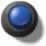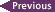# PHY138Y - Mechanics - Class 13 - Wednesday October 26, 2005

## Introduction

"Music is a hidden practice of the soul, which does not know that it is doing mathematics."

Leibniz

## Test Review

 The class on Monday, October 31, will be a review for the test. It will be all PowerPoint based. You may access a pdf of the PowerPoint with the button to the right.## Today's Class

We finished all parts of Chapter 13 that are part of the syllabus for this course.

## Angular Velocity Vector

 So far whenever we have discussed circular motion we have ignored the factor fact that the angular velocityis a vector, which is why we call it the velocity instead of the speed. We have used a sign convention that counter-clockwise rotations are positive and clockwise rotations are negative. Thus, if you are standing to the right of the rotating object the angular velocity is positive. If you are standing to the left of the object it is negative.

We have gotten pretty far with this sign convention. But now it is time to account for the fact that the angular velocity really is a vector, with both a magnitude and a direction. In what direction can it point?

There is only one symmetry of the rotating wheel: the axis of rotation. So it is reasonable to say that the angular velocity vector points along that axis. But does it point to the right or the left? There is a convention to decide this:

Have the fingers of your right hand curl in the direction of the rotation. Then your thumb points in the direction of the angular velocity vector.

 A Flash animation of this "right hand screw rule" is available via the button to the right.The animation to the right shows the angular velocity vector for our rotating object. You may find it helpful to recall the r-t-z coordinate system the text introduced for describing circular motion in §7.2; we discussed this in Class 7 on October 3. The angular velocity vector points in the z direction of this coordinate system.

## Supplementary Notes

 We discussed forces on the hip. Supplementary Notes in pdf format are available on this topic.## In-Class Questions

 We asked this question in class. The correct answer is 3: the meter stick has a mass of 1 kg. Initially, about 40% of the class answered 2, and only a few answered 5. The number of people answering 1, 3, or 4 was about the sameAfter some in-class discussion, about 60% of the class got the right answer and about 40% answered 4 with only a handful of other responses.

 We also asked this question. The correct answer, as we showed by doing a demonstration, is 2: the sliding mass gets down the ramp the fastest. About 60% of the class got this answer before doing the demonstration.## Class Materials

 Pdf version of the PowerPoint on the side screens.Today's Journal.## Suggested Problem

In the first In-Class Question about the meter stick with a 1 kg mass hanging from one end, show that you get the same answer for the mass of the meter stick, 1kg, when you analyse the total torques and forces acting:

• About the left-hand side of the meter stick
• About the right-hand side of the meter stickThe arrows let you jump to the previous/next class summaries.